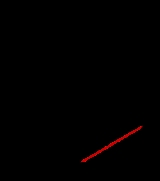xChord (geometry)Overview

A chord of a circle
Circle
A circle is a simple shape of Euclidean geometry consisting of those points in a plane that are a given distance from a given point, the centre. The distance between any of the points and the centre is called the radius....

is a geometric
Geometry
Geometry arose as the field of knowledge dealing with spatial relationships. Geometry was one of the two fields of pre-modern mathematics, the other being the study of numbers ....

line segment
Line segment
In geometry, a line segment is a part of a line that is bounded by two end points, and contains every point on the line between its end points. Examples of line segments include the sides of a triangle or square. More generally, when the end points are both vertices of a polygon, the line segment...

whose endpoints both lie on the circumference
Circumference
The circumference is the distance around a closed curve. Circumference is a special perimeter.-Circumference of a circle:The circumference of a circle is the length around it....

of the circle.
A secant or a secant line
Secant line
A secant line of a curve is a line that intersects two points on the curve. The word secant comes from the Latin secare, to cut.It can be used to approximate the tangent to a curve, at some point P...

is the line extension of a chord. More generally, a chord is a line segment joining two points on any curve, such as but not limited to an ellipse. A chord that passes through the circle's center point is the circle's diameter.
Among properties of chords of a circle
Circle
A circle is a simple shape of Euclidean geometry consisting of those points in a plane that are a given distance from a given point, the centre. The distance between any of the points and the centre is called the radius....

are the following:
1. Chords are equidistant from the center only if their lengths are equal.
2. A chord's perpendicular bisector passes through the centre.
3. If the line extensions (secant lines) of chords AB and CD intersect at a point P, then their lengths satisfy AP·PB = CP·PD (power of a point theorem).

The area that a circular chord "cuts off" is called a circular segment
Circular segment
In geometry, a circular segment is an area of a circle informally defined as an area which is "cut off" from the rest of the circle by a secant or a chord. The circle segment constitutes the part between the secant and an arc, excluding the circle's center...

.
The midpoints of a set of parallel chords of an ellipse are collinear
Collinearity
A set of points is collinear if they lie on a single line. Related concepts include:In mathematics:...

.
Chords were used extensively in the early development of trigonometry
Trigonometry
Trigonometry is a branch of mathematics that studies triangles and the relationships between their sides and the angles between these sides. Trigonometry defines the trigonometric functions, which describe those relationships and have applicability to cyclical phenomena, such as waves...

.